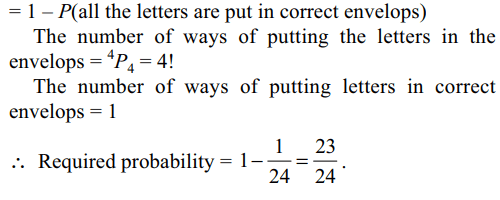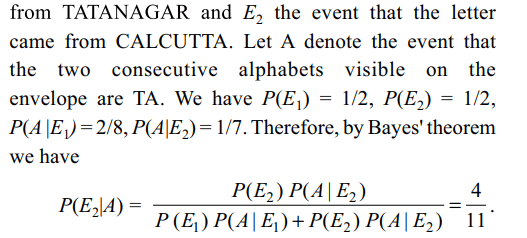## Probability Questions and Answers Part-4

1. Each of two persons A and B toss three fair coins. The probability that both get the same number of heads is
a) $\frac{3}{8}$
b) $\frac{1}{9}$
c) $\frac{5}{16}$
d) $\frac{7}{16}$

Explanation: Let X be the number of heads obtained by A and Y be the number of heads obtained by B. Note that both X and Y are binomial variate with parameters n = 3 and p = 1/2.2. Three boys and two girls stand in a queue. The probability, that the number of boys ahead of every girl is at least one more than the number of girls ahead of her, is
a) $\frac{1}{2}$
b) $\frac{1}{3}$
c) $\frac{2}{3}$
d) $\frac{3}{4}$

Explanation: Let E denote the event that the number of boys ahead of every girl is at least one more than the number of girls ahead of her. Note that E' consists of the following arrangements.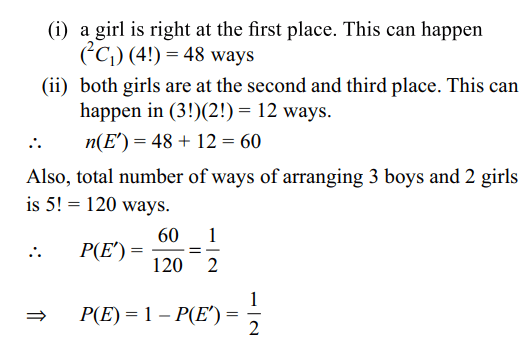3. Six distinct numbers are selected from first 150 natural number. The probability all the six numbers are divisible both by 3 and 5 is
a) 1/33
b) 1/35
c) 1/49
d) none of these

Explanation: Total number of ways of selecting 6 numbers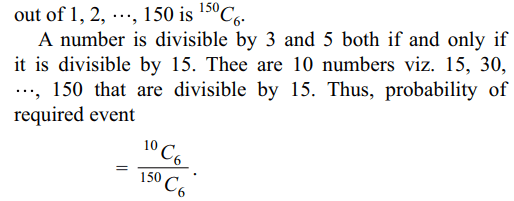4. There are two balls in an urn whose colours are not known (each ball can be either white or black). A white ball is put into the urn. A ball is drawn from the urn. The probability that it is white is
a) 1/4
b) 1/3
c) 2/3
d) 1/6

Explanation: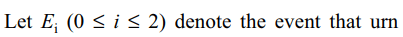5. A natural number x is chosen at random from the first one hundred natural numbers. The probability that $\frac{\left(x-20\right)\left(x-40\right)}{x-30} < 0$
is
a) 1/50
b) 3/50
c) 3/25
d) 7/25

Explanation: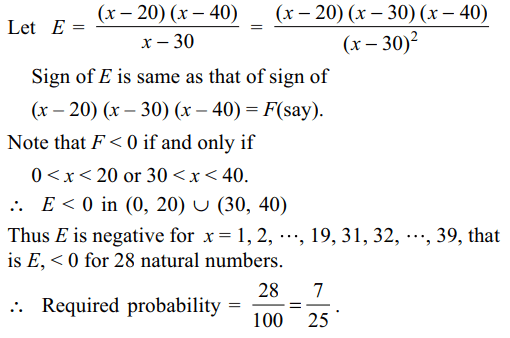6. An experiment has 10 equally likely outcomes. Let A and B be two non-empty events of the experiment. If A consists of 4 outcomes, the number of outcomes that B must have so that A and B are independent, is
a) 2, 4 or 8
b) 3, 6 or 9
c) 4 or 8
d) 5 or 10.

Explanation:7. If A, B and C are three events such that $P\left(B\right)=\frac{3}{4},P\left(A\cap B\cap C' \right)=\frac{1}{3}$       and $P\left(A'\cap B\cap C' \right)=\frac{1}{3}$    , then $P\left( B\cap C \right)$   is equal to
a) $\frac{1}{12}$
b) $\frac{1}{6}$
c) $\frac{1}{15}$
d) $\frac{1}{9}$

Explanation: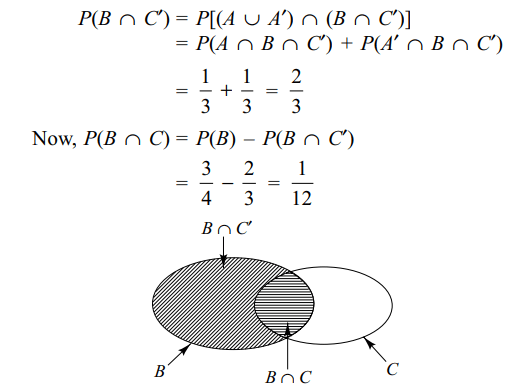8. A fair coin is tossed n times. If the probability that head occurs 6 times is equal to the probability that head occurs 8 times, then value of n is
a) 24
b) 48
c) 14
d) 16

Explanation: Let X be the number of times head occurs.9. A person writes 4 letters and 4 addresses on 4 envelopes. If the letters are placed in the envelopes at random, the probability that not all letters are placed in correct envelopes is
a) 1/24
b) 11/24
c) 5/8
d) 23/24